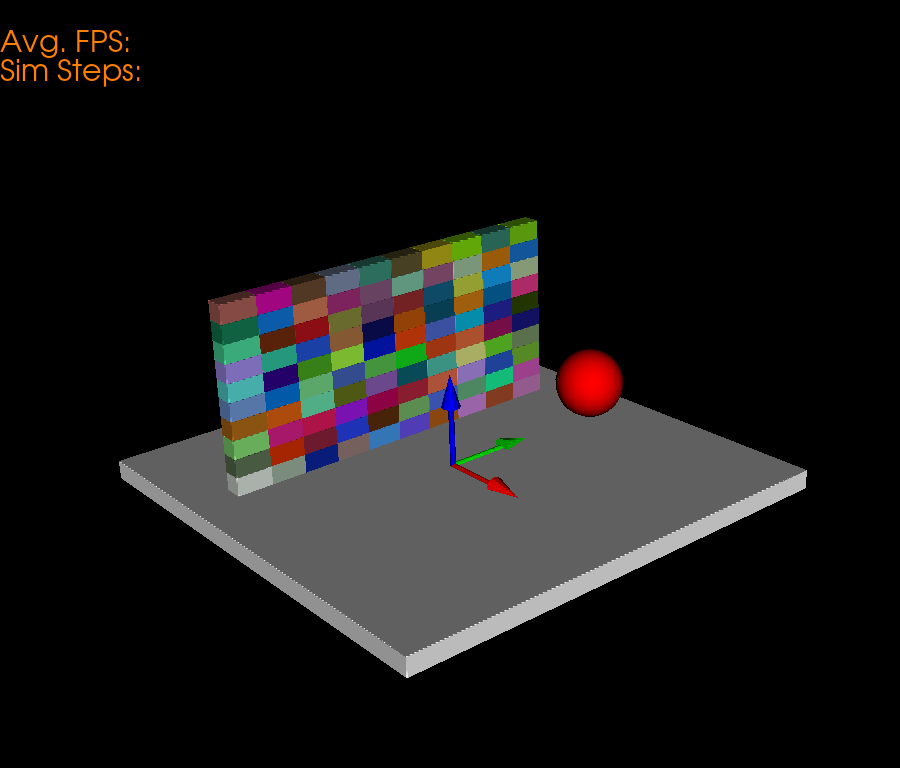# Brick Wall Simulation¶

This example simulation shows how to use pybullet to render physics simulations in fury. In this example we specifically render a ball beign thrown at a brick wall.

First some imports.

```import numpy as np
from fury import window, actor, ui, utils
import itertools
import pybullet as p
```

Next, we initialize a pybullet client to render the physics. We use DIRECT mode to initialize pybullet without a GUI.

```p.connect(p.DIRECT)
```

Apply gravity to the scene. In pybullet all values are in SI units.

```p.setGravity(0, 0, -10)
```

We define some global parameters so that its easier for us to tweak the tweak the simulation.

```# Ball Parameters
ball_color = np.array([1, 0, 0])
ball_mass = 3
ball_position = np.array([2, 0, 1.5])
ball_orientation = np.array([0, 0, 0, 1])

# Base Plane Parameters
base_size = np.array([5, 5, 0.2])
base_color = np.array([1, 1, 1])
base_position = np.array([0, 0, -0.1])
base_orientation = np.array([0, 0, 0, 1])

# Wall Parameters
wall_height = 10
wall_width = 10
brick_mass = 0.5
brick_size = np.array([0.2, 0.4, 0.2])
```

Now we define the required parameters to render the Ball.

```# Ball actor
ball_actor = actor.sphere(centers=np.array([[0, 0, 0]]),
colors=ball_color,

# Collision shape for the ball.
ball_coll = p.createCollisionShape(p.GEOM_SPHERE,

# Creating a multi-body which will be tracked by pybullet.
ball = p.createMultiBody(baseMass=3,
baseCollisionShapeIndex=ball_coll,
basePosition=ball_position,
baseOrientation=ball_orientation)

# Change the dynamics of the ball by adding friction and restitution.
p.changeDynamics(ball, -1, lateralFriction=0.3, restitution=0.5)
```

Render a base plane to support the bricks.

```base_actor = actor.box(centers=np.array([[0, 0, 0]]),
directions=[0, 0, 0],
scales=base_size,
colors=base_color)

base_coll = p.createCollisionShape(p.GEOM_BOX,
halfExtents=base_size / 2)
# half of the actual size.

base = p.createMultiBody(
baseCollisionShapeIndex=base_coll,
basePosition=base_position,
baseOrientation=base_orientation)

p.changeDynamics(base, -1, lateralFriction=0.3, restitution=0.5)
```

Now we render the bricks. All the bricks are rendered by a single actor for better performance.

```nb_bricks = wall_height * wall_width

brick_centers = np.zeros((nb_bricks, 3))

brick_directions = np.zeros((nb_bricks, 3))
brick_directions[:] = np.array([1.57, 0, 0])

brick_orns = np.zeros((nb_bricks, 4))

brick_sizes = np.zeros((nb_bricks, 3))
brick_sizes[:] = brick_size

brick_colors = np.random.rand(nb_bricks, 3)

brick_coll = p.createCollisionShape(p.GEOM_BOX,
halfExtents=brick_size / 2)

# We use this array to store the reference of brick objects in pybullet world.
bricks = np.zeros(nb_bricks, dtype=np.int8)

# Logic to position the bricks appropriately to form a wall.
i = 0
for k in range(wall_height):
for j in range(wall_width):
center_pos = np.array([-1, (j * 0.4) - 1.8, (0.2 * k) + 0.1])
brick_centers[i] = center_pos
brick_orns[i] = np.array([0, 0, 0, 1])
bricks[i] = p.createMultiBody(baseMass=brick_mass,
baseCollisionShapeIndex=brick_coll,
basePosition=center_pos,
baseOrientation=brick_orns[i])
p.changeDynamics(bricks[i], -1, lateralFriction=0.1, restitution=0.1)
i += 1

brick_actor = actor.box(centers=brick_centers,
directions=brick_directions,
scales=brick_sizes,
colors=brick_colors)
```

Now, we define a scene and add actors to it.

```scene = window.Scene()

# Create show manager.
showm = window.ShowManager(scene,
size=(900, 768), reset_camera=False,
order_transparent=True)

showm.initialize()

# Counter iterator for tracking simulation steps.
counter = itertools.count()

# Variable for tracking applied force.
apply_force = True
```

Now, we define methods to sync objects between fury and Pybullet.

```# Get the position of base and set it.
base_pos, _ = p.getBasePositionAndOrientation(base)
base_actor.SetPosition(*base_pos)

# Do the same for ball.
ball_pos, _ = p.getBasePositionAndOrientation(ball)
ball_actor.SetPosition(*ball_pos)

# Calculate the vertices of the bricks.
vertices = utils.vertices_from_actor(brick_actor)
num_vertices = vertices.shape
num_objects = brick_centers.shape
sec = int(num_vertices / num_objects)
```

# Syncing Bricks¶

Here, we perform three major steps to sync bricks accurately. * Get the position and orientation of the bricks from pybullet. * Calculate the Rotation Matrix.

• Get the difference in orientations (Quaternion).

• Generate the corresponding rotation matrix according to that difference.

• Reshape it in a 3x3 matrix.

• Perform calculations to get the required position and orientation.

• Update the position and orientation.

```def sync_brick(object_index, multibody):
pos, orn = p.getBasePositionAndOrientation(multibody)

rot_mat = np.reshape(
p.getMatrixFromQuaternion(
p.getDifferenceQuaternion(orn, brick_orns[object_index])),
(3, 3))

vertices[object_index * sec: object_index * sec + sec] = \
(vertices[object_index * sec: object_index * sec + sec] -
brick_centers[object_index]) @ rot_mat + pos

brick_centers[object_index] = pos
brick_orns[object_index] = orn
```

A simpler but inaccurate approach is used here to update the position and orientation.

```def sync_actor(actor, multibody):
pos, orn = p.getBasePositionAndOrientation(multibody)
actor.SetPosition(*pos)
orn_deg = np.degrees(p.getEulerFromQuaternion(orn))
actor.SetOrientation(*orn_deg)
```

Here, we define a textblock to display the Avg. FPS and simulation steps.

```fpss = np.array([])
tb = ui.TextBlock2D(text="Avg. FPS: \nSim Steps: ", position=(0, 680),
font_size=30, color=(1, 0.5, 0))
```

Set the camera for better visualization.

```scene.set_camera(position=(10.46, -8.13, 6.18),
focal_point=(0.0, 0.0, 0.79),
view_up=(-0.27, 0.26, 0.90))
```

Timer callback is created which is responsible for calling the sync and simulation methods.

```# Create timer callback which will execute at each step of simulation.
def timer_callback(_obj, _event):
global apply_force, fpss
cnt = next(counter)
showm.render()

if cnt % 1 == 0:
fps = scene.frame_rate
fpss = np.append(fpss, fps)
tb.message = "Avg. FPS: " + str(np.round(np.mean(fpss), 0)) + \
"\nSim Steps: " + str(cnt)

# Get the position and orientation of the ball.
ball_pos, ball_orn = p.getBasePositionAndOrientation(ball)

# Apply force for 5 times for the first step of simulation.
if apply_force:
# Apply the force.
p.applyExternalForce(ball, -1,
forceObj=[-10000, 0, 0],
posObj=ball_pos,
flags=p.WORLD_FRAME)
apply_force = False

# Set position and orientation of the ball.
sync_actor(ball_actor, ball)

# Updating the position and orientation of each individual brick.
for idx, brick in enumerate(bricks):
sync_brick(idx, brick)
utils.update_actor(brick_actor)

# Simulate a step.
p.stepSimulation()

# Exit after 2000 steps of simulation.
if cnt == 130:
showm.exit()

# Add the timer callback to showmanager.
# Increasing the duration value will slow down the simulation.

interactive = False

# start simulation
if interactive:
showm.start()

window.record(scene, out_path="viz_brick_wall.png", size=(900, 768))
```Total running time of the script: ( 0 minutes 0.211 seconds)

Gallery generated by Sphinx-Gallery